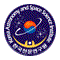# East Asian VLBI Network (EAVN)

## Angular Resolution and Largest Detectable Angular Scale

For KaVA, the expected angular resolutions for the K band and the Q band are about 1.2 mas and 0.6 mas, respectively. The synthesized beam size strongly depends on UV coverage, and could be higher than the values mentioned above because the baselines projected on UV plane become shorter than the distance between antennas. The beam size can be calculated approximately by the following formula;(1)

where λ and Β are observed wavelength in centimeter and the maximum baseline length in kilometer, respectively.

The minimum detectable angular scale for interferometers can be also expressed by equation (1), where the baseline length B is replaced with the shortest one among the array. Because of the relatively short baselines provided by KVN, ~300 km, KaVA is able to detect an extended structure up to 9 mas and 5 mas for the K and Q bands, respectively.

As for an EAVN array which adds Tianma/NRO45 to KaVA, the longest/shortest baselines remain the same as those of KaVA, so the maximum angular resolutions and the largest detectable angular scales are basically the same, although their detailed values in a synthesized image are dependent on the scheme of UV weighting as well as the UV coverage.EAVN ( East Asia VLBI Network )